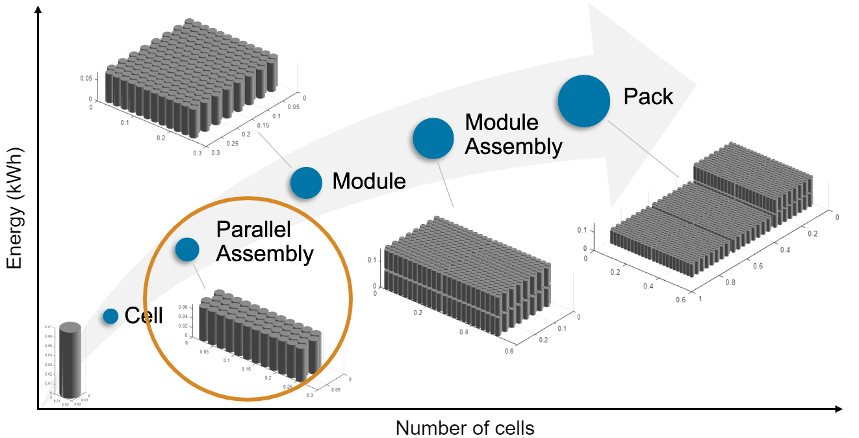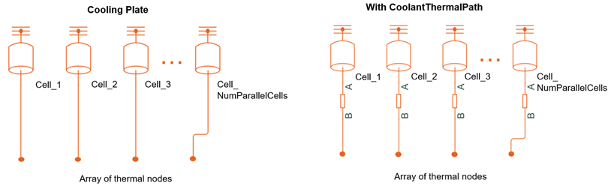# ParallelAssembly

Create parallel assembly of battery cells

## Description

Use `ParallelAssembly` to create a battery parallel assembly object that represents a number of cells connected electrically in parallel under a specific topological configuration or geometrical arrangement. You can use this object as an input to the `Module` object.

To specify the number of cells connected in parallel, use the `NumParallelCells` property. To generate a Simscape™ model of your `ParallelAssembly` object, use the `buildBattery` function. This object only supports the definition of structural or design parameters. You can modify the run-time parameters for this model block and its constituent cells after you create the model.

The `ParallelAssembly` object is the second stage of a battery pack system model in a bottom-up approach. Pack models are required for architecture evaluation in early development stages, software and hardware development, system integration and requirement evaluation, cooling system design, control strategy development hardware-in-the-loop, and many more applications.To meet battery system packaging and space requirements, you can arrange the battery cells in many different topologies or geometrical configurations. Use the `Topology` property of the `ParallelAssembly` object to define the geometrical arrangement of your battery in a 3-D Cartesian coordinate system.

The topology of the parallel assembly depends on the format of the battery cells that comprise the assembly. Depending on the value of the `Topology` property, you can modify the `Rows` property to best represent your specific design. The `Rows` property is defined with respect to the x-axis of the Cartesian coordinate system. By default, the cells are stacked along the y-axis.

This figure shows the different topologies of a parallel assembly according to the format of the battery cells that comprise the assembly:To modify the number of cells connected in parallel, use the `NumParallelCells` property. You can modify the topology of the parallel assembly by using the `Topology` property, which depends on the cell format. The `Rows` property is defined with respect to the x-axis of the Cartesian system. By default, cells are stacked along the y-axis.

## Creation

### Syntax

``````import simscape.battery.builder.*; batteryParallelAssembly = ParallelAssembly``````
``````import simscape.battery.builder.*; batteryParallelAssembly = ParallelAssembly(Name=Value)``````

### Description

``````import simscape.battery.builder.*; batteryParallelAssembly = ParallelAssembly``` creates a parallel assembly that comprises battery cells with default property values.```

example

``````import simscape.battery.builder.*; batteryParallelAssembly = ParallelAssembly(Name=Value)``` sets Properties using one or more name-value arguments. For example, create a parallel assembly with 48 cylindrical cells stacked in a square topology over four rows with an intercell gap equal to 0.001 m.parallelAssembly = ParallelAssembly(... NumParallelCells=48, ... Cell=Cell(Geometry=CylindricalGeometry), ... Topology="Square", ... Rows=4, ... InterCellGap=simscape.Value(0.001,"m"));```

## Properties

expand all

Cell component in the parallel assembly, specified as a `Cell` object. The `ParallelAssembly` object creates this component and then electrically connects it in parallel a number of times equal to the value of the `NumParallelCells` property.

Example: ```batteryParallelAssembly.Cell = Cell(Geometry=CylindricalGeometry)```

Number of cells connected in parallel inside the parallel assembly, specified as a strictly positive and finite integer. The value of this property must be less than `150`.

Example: `batteryParallelAssembly.NumParallelCells = 48`

Number of rows of the parallel assembly relative to the stacking axis, specified as a strictly positive integer. The value of this property must be less than `50` and less than the value of the `NumParallelCells` property.

Example: `batteryParallelAssembly.Rows = 4`

Geometrical arrangement of the cells relative to the cell format, specified as either `"Square"` or `"Hexagonal"` for cylindrical cells, `"SingleStack"` or `"NStack"` for prismatic cells, or `"SingleStack"` for pouch cells.

Example: `batteryParallelAssembly.Topology = "Hexagonal"`

Model resolution or fidelity in the simulation, specified as:

• `"Lumped"` — Choose this value for the lowest fidelity. The assembly uses only one electrical model with parameters scaled up according to the number of cells in series and in parallel. To obtain the fastest model compilation and running time, set the model resolution to this value.One cell model block represents all the cell components inside the orange box. The object scales the electrical parameters of the cell model blocks by using the `NumParallelCells` property.

• `"Detailed"` — Choose this value for the highest fidelity. The assembly uses one electrical model and one thermal model for each battery cell in the parallel assembly.A number of cell model blocks equal to the value of the `NumParallelCells` property represents each cell component.

Example: `batteryParallelAssembly.ModelResolution = "Detailed"`

State-of-charge balancing strategy for the parallel assembly, specified as `""` or `"Passive"`. Set this property to `"Passive"` to add an ideal balancing circuit connected in parallel to the `ParallelAssembly` Simscape model and a physical port used for switch control. The switch is open when the control signal is equal to 0. The switch is closed when the control signal is equal to 1.Example: ```batteryParallelAssembly.BalancingStrategy = "Passive"```

Option to use a simple thermal resistance block connected between the cells and a Simscape thermal network, specified as `"CellBasedThermalResistance"` or `""`.Example: ```batteryParallelAssembly.AmbientThermalPath = "CellBasedThermalResistance"```

Option to use a simple thermal resistance block connected between the cells and a Simscape thermal network, specified as `"CellBasedThermalResistance"` or `""`.

If you also define a cooling plate surface, the object connects the thermal resistance block between each battery thermal model and its corresponding element in the array of thermal nodes. You can use this thermal resistance to capture conductive and convective heat transfer mechanisms related to the cell and cooling system design.Example: ```batteryParallelAssembly.CoolantThermalPath = "CellBasedThermalResistance"```

Option to use a cooling plate component at a specific surface boundary, specified as `"Top"`, `"Bottom"`, or `""`.

This option adds a vectorized array of thermal nodes connector to the `ParallelAssembly` object. The object connects each element of the array of thermal nodes to each thermal model. If you also select a coolant thermal path, the object connects a Thermal Resistance block between each battery thermal model and its corresponding element in the array of thermal nodes. If you define a cell geometry, the `ThermalNodes` property contains the number of thermal nodes, their dimensions, and their locations in a 2-D Cartesian plane.Example: `batteryParallelAssembly.CoolingPlate = "Top"`

Shortest distance between the cells inside the parallel assembly, specified as a positive scalar or a `simscape.Value` object that represents a positive scalar with a unit of length. The value of this property must be less than 0.1 m.

If you set this property directly with a positive scalar value instead of using a `simscape.Value` object, the object converts the value to a `simscape.Value` object with meter as its physical unit.

Example: ```batteryParallelAssembly.InterCellGap = simscape.Value(0.01,"m")```

Example: `batteryParallelAssembly.InterCellGap = 0.01`

Location of the battery object in a 3-D Cartesian coordinate system, specified as a vector of real and finite entries.

Example: `batteryParallelAssembly.Position = [0 0 0]`

Name of the parallel assembly, specified as a string.

Example: `batteryParallelAssembly.Name = "ParallelAssembly2"`

Additional non-cell-related mass added to the parallel assembly by components such as busbars, tabs, and collector plates, specified as a strictly positive double greater than or equal to 1.

Example: `batteryParallelAssembly.MassFactor = 1.2`

Preferential stacking direction for the arrangement of battery cells in a 2-D Cartesian coordinate system, specified as either `"X"` or `"Y"`.

This figure shows the global coordinate system for batteries.To plot the parallel assembly object in the direction of the x-axis, set this property to `"X"` before creating the `BatteryChart` object.

Example: `batteryParallelAssembly.StackingAxis = "Y"`

Option to use electrical resistance blocks to represent additional electrical resistances from non-cell components, specified as `"No"` or `"Yes"`.

Example: `batteryParallelAssembly.NonCellResistance = "Yes"`

Volume of the battery, returned as a `simscape.Value` object with a unit of volume.

Cumulative mass of the battery, returned as a `simscape.Value` object with a unit of mass.

Number of cell model blocks in the simulation, returned as a double.

Numbering for all of the cells inside of the battery, returned as a structure.

Vectorized thermal node information for external boundary conditions, returned as a structure. This information comprises the XY location, XY dimensions, and number of thermal nodes. If you do not define a battery cell geometry, this property is an empty structure.

Type of battery object, returned as `"ParallelAssembly"`.

## Examples

collapse all

Create a `Cell` object with a pouch geometry.

`batteryCell = Cell(Geometry=PouchGeometry)`

Create a `ParallelAssembly` object of three cells with the default topology.

`pSet = ParallelAssembly(Cell=batteryCell,NumParallelCells=3)`

Create a `Cell` object with a cylindrical geometry.

`batteryCell = Cell(Geometry=CylindricalGeometry)`

Create a `ParallelAssembly` object of 46 cells stacked in 14 rows .

`pSet = ParallelAssembly(Cell=batteryCell,NumParallelCells=46,Rows=14)`

Set the topology of the parallel assembly to `"Square"`.

`pSet.Topology = "Square";`

Visualize the parallel assembly by using a `BatteryChart` object.

`pSetChart = BatteryChart(Battery=pSet)`

## Version History

Introduced in R2022b